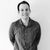# Automating date calculation in the Field Calculator

688
5
06-15-2021 06:46 AM
Labels (3)New Contributor III

Good afternoon all!

I am trying to automate date calculations using the field calculator in ArcGIS Pro.

I have defined survey dates, which I would like to use to create a reinspection dates.

The reinspection date is 1 year for high risk zones, 3 years for medium risk zone and 5 years for low risk zones.

Is there a piece of code I could use in the field calculator to automate this calculation?

The best sample I came out with for high risk zones is:

Expression:
arcpy.time.ParseDateTimeString(!SurveyDate!) + datetime.timedelta(days=365)

However, this did not work when I tried to execute the script.

Any help is appreciated.

Thank you

Tags (5)
2 Solutions

Accepted SolutionsbyMVP Frequent Contributor

edit - I can see this same post was answered in Python questions.byMVP Frequent Contributor

I'd post any continuations as new posts to prevent threads becoming a Q and A or bugfixing session.

``````#Expression line:
reclass(!SurveyDate!, !RiskZone!)

#Code block:
def reclass(SurveyDate, RiskZone):
if (RiskZone == 'LOW'):
return SurveyDate + datetime.timedelta(days=486)
elif (RiskZone == 'MED'):
return  SurveyDate + datetime.timedelta(days=1095)
elif (RiskZone = 'HIGH'):
return SurveyDate + datetime.timedelta(days=1825)``````
5 RepliesbyMVP Frequent Contributor

edit - I can see this same post was answered in Python questions.New Contributor III

Thank you David - I asked in both communities as I didn't know which was more relevant :)New Contributor III

I am now trying to add in the risk zones and my statement does not seem to be working.

Field Calculator:

``````Expression line:
reclass(!SurveyDate!)

Code block:
def reclass(SurveyDate):
if (RiskZone = LOW):
return "SurveyDate + datetime.timedelta(days=486)"
elif (RiskZone = MED):
return return "SurveyDate + datetime.timedelta(days=1095)"
elif (RiskZone = HIGH):
return return "SurveyDate + datetime.timedelta(days=1825)"``````

Attached is the syntax error.

Any assistance is appreciated.byMVP Frequent Contributor

I'd post any continuations as new posts to prevent threads becoming a Q and A or bugfixing session.

``````#Expression line:
reclass(!SurveyDate!, !RiskZone!)

#Code block:
def reclass(SurveyDate, RiskZone):
if (RiskZone == 'LOW'):
return SurveyDate + datetime.timedelta(days=486)
elif (RiskZone == 'MED'):
return  SurveyDate + datetime.timedelta(days=1095)
elif (RiskZone = 'HIGH'):
return SurveyDate + datetime.timedelta(days=1825)``````New Contributor III

Thank you, will do that in future!